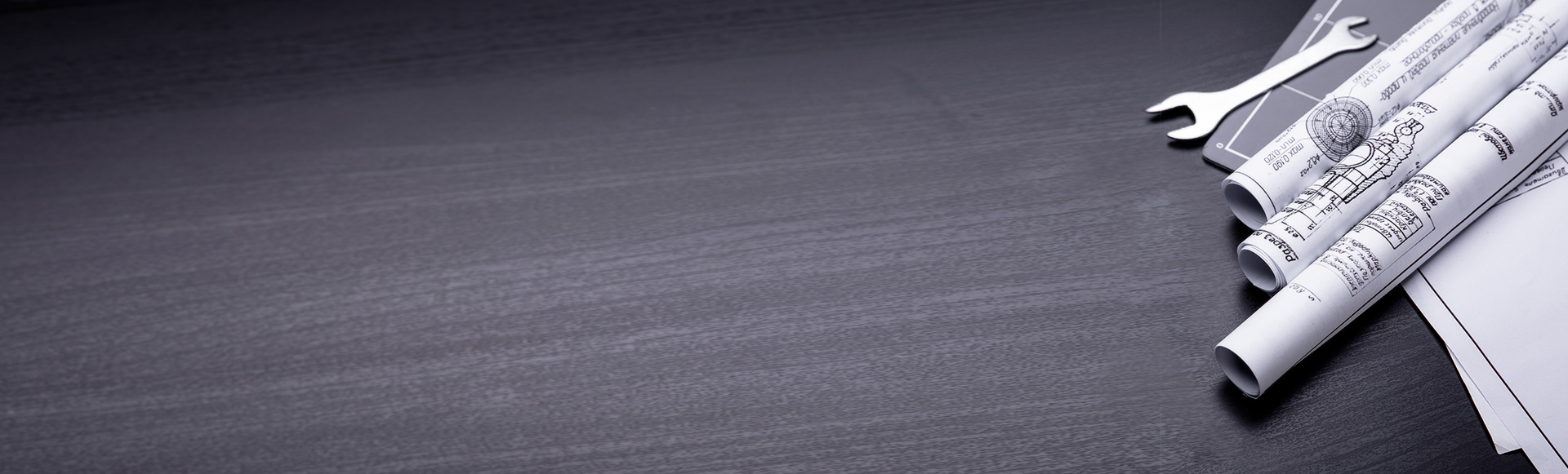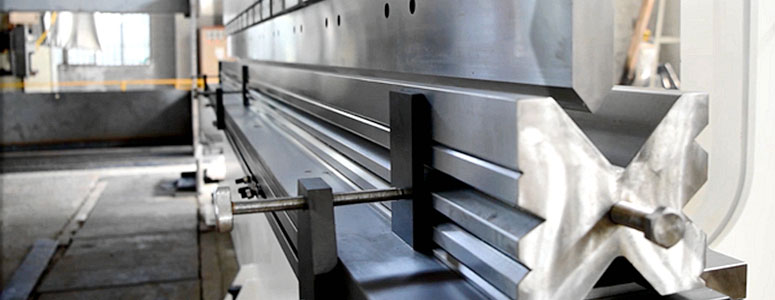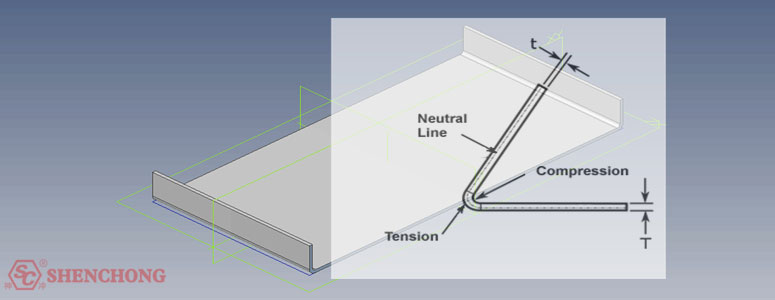MACHINE GUIDE
Position：
What is Press Brake Bend Allowance? [Tips for Beginners]
Time：2021-01-08
Visits：980

## 1. What is the press brake bend allowance?The bend allowance is used to measure the amount of additional material needed to accommodate the bending in the sheet metal part. It is a method used to calculate the unfolded length of the flat sheet metal part required to construct a specific radius and angle bend.

The calculation takes into account the bending angle, bending radius, material thickness, and other material properties (such as Y and K factors) to create the bend.

Bend allowance is a term that describes how much material is needed between two panels to accommodate a given bend.

Although the bend allowance is usually difficult to determine all cases, it is fairly easy to predict and calculate in many standard cases. Determining the bend allowance is often referred to as “bending development” or simply “development”.

The unfolded length calculation also compensates for the stretching in the bending area. When bending or forming metal parts, the material outside the neutral bending axis is usually stretched, and the material inside the neutral bending axis is compressed.

By establishing an appropriate material description and a formula for accurately calculating the unfolded length, this material characteristic can be automatically considered.

The precise unfolding length calculation can be used to capture the design intent in the solid model. And it can also develop an accurate flattened model that the manufacturer can use when manufacturing the actual product. Get in the habit of first determining how to calculate the expanded length.

- System default equation: Only Y or K factors are used to calculate the unfolding length.

- Provided bend table: Use predefined, standard bending tables to calculate the unfolded length.

- Customized bend table: Use the customized bending table in Pro/Table to calculate the unfolded length.

## 2. How to determine the bend allowance?The press brake bend allowance is used to calculate the bending in sheet metal manufacturing.

When a metal plate is bent, its total length will change due to the internal compression of the bending and the external tension. A line passing through the thickness of the bending is called the neutral axis.

When the part is bent, the length will not change. The material inside the wire is in a compressed state. The material outside the wire is in a stretched state.

The position of the neutral axis changes according to the bending angle and the bending radius.

The K factor is the ratio of the distance from the neutral line to the inner edge of the material to the thickness of the material.

The specific formula is as following:

Bend allowance = π/180*A*(IR+K*MT)

Note:

The A is the bending angle, IR is the inside bending radius, the T is the material thickness.

In general, the K factor is not less than 0.25, and it cannot be greater than 0.5, because it is physically impossible for the compression inside the bend to be higher than the tension outside.

Basically, the K factor is an indirect measure of the ratio of compression to extension in bending-the higher the K factor, the greater the compression. The K factor depends on the material used and the type of bending created.

Once the bend allowance is calculated, it is added to the required machining length to obtain the material length required to manufacture the bent part.

The machines commonly used to bend metal plates include brake presses (also called presses) and box and disc brakes.

Various methods of bending on each type of brake are used. Commonly used brake bending materials include aluminum and mild steel; certain types of ductile plastics can also be bent using these processing methods.

So, during the process of consulting the press brake, don’t forget to provide the relevant parameters to Shenchong’s tech team, then we will calculate the bend allowance for your CNC press brake with the most professional advice.